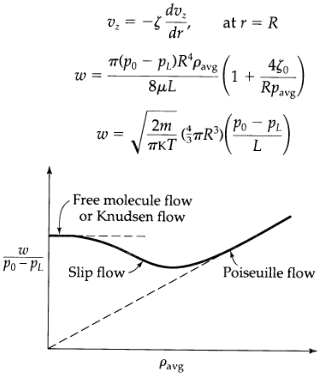Create an Account

Home / Questions / Low density phenomena in compressible tube flow2 3 Fig 2B9 As the pressure is decreased i...

Low density phenomena in compressible tube flow2 3 Fig 2B9 As the pressure is decreased in the system studied in Example 2 3 2 deviations from

Low-density phenomena in compressible tube flow2,3 (Fig. 2B.9). As the pressure is decreased in the system studied in Example 2.3-2, deviations from Eqs. 2.3-28 and 2.3-29 arise. The gas behaves as if it slips at the tube wall. It is conventional2 to replace the customary "no-slip" boundary condition that vz = 0 at the tube wall by in which ? is the slip coefficient. Repeat the derivation in Example 2.3-2 using Eq. 2B.9-1 as the boundary condition. Also make use of the experimental fact that the slip coefficient varies inversely with the pressure ? = ?0/p, in which ?0 is a constant. Show that the mass rate of flow is in which Pavg = ?1(p0 + p1) When the pressure is decreased further, a flow regime is reached in which the mean free path of the gas molecules is large with respect to the tube radius (Knudsen flow). In that regime3 in which m is the molecular mass and K is the Boltzmann constant. In the derivation of this result it is assumed that all collisions of the molecules with the solid surfaces are diffuse and not specular, the results in Eqs. 2.3-29, 2B.9-2, and 2B.9-3 are summarized in Fig. 2B.9.Jul 24 2020 View more View LessSubscribe To Get Solution